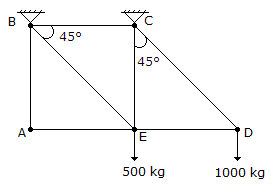# Civil Engineering - UPSC Civil Service Exam Questions

41.

A shaft turns at 150 rpm under a torque of 1500 Nm. Power transmitted is

 A. 15 π kW B. l0 π kW C. 7.5 π kW D. 5 π kW

Explanation:

No answer description available for this question. Let us discuss.

42.

Consider the following factors :
1. Initial prestress
2. Losses in prestress
3. Depth of cable from extreme compression fibre.
Those which affect the ultimate moment capacity of a prestressed concrete beam would include

 A. 1 and 2 B. 1 and 3 C. 1 alone D. 3 alone

Explanation:

No answer description available for this question. Let us discuss.

43.

If the stream function is ψ = 2 xy, then the velocity at a point (1, 2) is equal to

 A. 2 B. 4 C. 20 D. 16

Explanation:

No answer description available for this question. Let us discuss.

44.

The cantilever frame shown in the given figure is supported by vertical links at B and C and carries loads as shown. The force in the bar AE isA. 500 kg B. 1000 kg C. zero D. 2500 kg

Explanation:

No answer description available for this question. Let us discuss.

45.

Two piles, one a bored cast-in-situ pile and another a precast driven pile, both of same length and diameter are constructed in a loose sand deposit. If the bearing capacity of the bored pile is Q1, and that of the precast driven pile is Q2, then which of the following is correct ?

 A. Q1 > Q2 B. Q1 = Q2 C. Q1 < Q2 D. No specific comparison may hold good